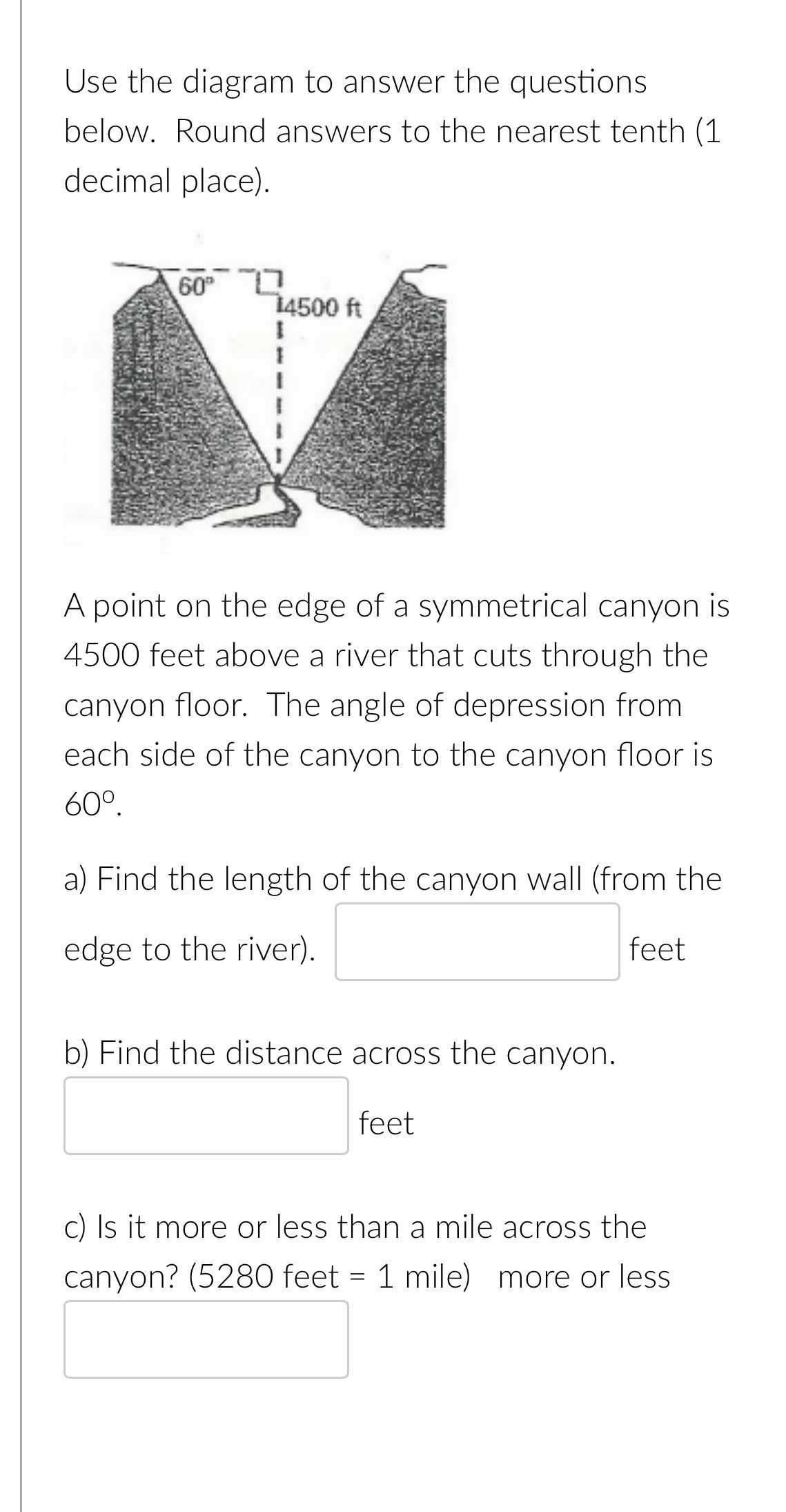### ¿Todavía tienes preguntas de matemáticas?

Pregunte a nuestros tutores expertos
Trigonometry
Pregunta

Use the diagram to answer the questions below. Round answers to the nearest tenth (1 decimal place). A point on the edge of a symmetrical canyon is$$4500$$ feet above a river that cuts through the canyon floor. The angle of depression from each side of the canyon to the canyon floor is

$$60 ^ { \circ }$$ . a) Find the length of the canyon wall (from the edge to the river). feet